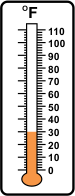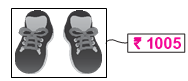SOF - IMO Level 2 Sample Papers for Class 3

Resources:

Class 3 sample paper & practice questions for the level 2 of International Mathematics Olympiad (IMO) are given below. Syllabus for level 2 is also mentioned for these exams. You can refer these sample paper & quiz for preparing for the exam.Sample Questions from Olympiad Success:

 Q.1 Q.2 Q.3 Q.4 Q.5 Q.6 Q.7 Q.8 Q.9 Q.10
 Q.1 Which is the closest temperature shown in the image given below?a) 45°F b) 28°F c) 20°F d) 15°F
 Q.2 Choose the correct option: 12 thousands + 12 hundreds + 12 tens + 12 = ________. a) 12,228 b) 13,332 c) 12,252 d) 13,308
 Q.3 There are 17 cartons, each containing 172 apples. Five cartons contain all the good apples. The remaining cartons are half-filled with good apples. How many bad apples are there in all? (IMO L2 2013) a) 1203 b) 1032 c) 1320 d) 1302
 Q.4 Choose the correct option: 50 hundreds - 50 tens = ______ a) 450 b) 4500 c) 4950 d) 4995
 Q.5 There are 14 cubes in one packet and the cost of one packet is Rs. 90. What is the cost of one cube? a) Rs. 7 b) Rs. 6.75 c) Rs. 6 d) Rs. 6.42
 Q.6 From 25,000, subtract the sum of 4,500 and 6,520: a) 20,500 b) 18,480 c) 21,000 d) 13,980
 Q.7 Sanchi has twice as many marbles as Sia. Jiya has thrice as many marbles as Sanchi. they have  81 marbles altogether.  How many marbles does Jiya have?  (IMO L2 2013) a) 54 b) 27 c) 18 d) 18
 Q.8 Muskaan has a wire of length 1274 cm. If this wire has covered with 5 equal sides, then what is the length that each side will take? a) 25 m 78 cm b) 1 m 27.4 cm c) 127 m 4 cm d) 2 m 54.8 cm
 Q.9 Mohit is thinking of a two-digit number. The digit in the one’s place is 4 more than that in the ten’s place. Given that the digit in the ten’s place is 7 less than the largest 1-digit number, what is the number that Mohit is thinking of?    (IMO L2 2017) a) 26 b) 62 c) 24 d) 42
 Q.10 Beena had Rs. 180. Her father gave her Rs. 500. How much more money should she save to buy the pair of shoes shown below? (IMO L2 2012)a) Rs. 525 b) Rs. 125 c) Rs. 425 d) Rs. 325